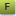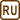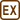## Electron and optical microscope

Reachable resolution power of the microscope is given by the wavelength of the used particles. This means that the smallest detail that we can observe has approximately the size of the wavelength. Let’s assume we want to see inside the atom which has a diameter of approximately 100 pm, this means we want to see details of approximately 10 pm.

a) What energy must the electrons have in the case we want to use electron microscope?

b) What energy must the photons have in the case we want to use optical microscope?

c) Which microscope is more practical and why?

• #### Hint

We have the de Broglie formula Broglieho vztahuconnecting the wavelength and momentum of the particle given by

$\lambda=\frac{h}{p} ,$

where h = 6.63·10−34 Js is Planck’s constant.

Energy of the photonis proportional to its frequency; therefore, not proportionate to its wavelength.

• #### Notation

 λ = 10 pm = 10−11 m wavelength Ee = ? (eV) energy of the electron Ef = ? (eV) energy of the photon

From tables:

 h = 6.63·10−34 Js Planck’s constant me = 9.11·10−31 kg electron mass c = 3.00·108 m s−1 speed of light in vacuum
• #### Solution

a) Momentum pe and the wavelength λe of the electron are related by de Broglie formula:

$\lambda=\frac{h}{p_{\mathrm{e}}} ,$

where h = 6.63·10−34 Js is Planck’s constant.

We can determine the kinetic energy of the electron Ee from its momentum pe

$E_{\mathrm{e}}=\frac{p_{\mathrm{e}}^2}{2m_{\mathrm{e}}}=\frac{\left(\frac{h}{\lambda_{\mathrm{e}}}\right)^2}{2m_{\mathrm{e}}}=\frac{h^2}{2m_{\mathrm{e}}\lambda_{\mathrm{e}}^2}=\frac{\left(6.63{\cdot}10^{-34}\right)^2}{2{\cdot}9.11{\cdot}10^{-31}\cdot\left(10^{-11}\right)^2}\,\mathrm{J}=2.4{\cdot}10^{-15}\,\mathrm{J}=15\,\mathrm{keV}.$

b) Energy of the photon Ef is equal to

$E_f=hf=h\frac{c}{\lambda}=\frac{6.63{\cdot}10^{-34}\cdot3.00{\cdot}10^8}{10^{-11}}\,\mathrm{J}=1.99{\cdot}10^{-14}\,\mathrm{J}=124\,\mathrm{keV} .$

c) In terms of more resolution, the electron microscope is more practical – it is sufficient to accelerate the electrons by voltage of 1.5 kV which is not so big. In optical microscope we would need to use gamma radiation (see electromagnetical spectra) which is hard to obtain and dangerous.